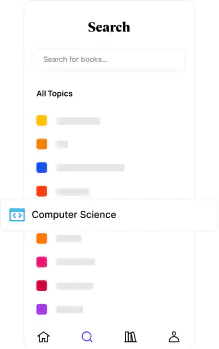📖[PDF] Regression Analysis with R by Giuseppe Ciaburro | Perlego
Start your free trial today and explore our endless library.📖 Book - PDF

# Regression Analysis with R

Giuseppe CiaburroShare book422 pagesEnglishePUB (mobile friendly) and PDFAvailable on iOS & Android
📖 Book - PDF

# Regression Analysis with R

Giuseppe Ciaburro
Book details
Citations

Build effective regression models in R to extract valuable insights from real data

Key Features

• Implement different regression analysis techniques to solve common problems in data science - from data exploration to dealing with missing values
• From Simple Linear Regression to Logistic Regression - this book covers all regression techniques and their implementation in R
• A complete guide to building effective regression models in R and interpreting results from them to make valuable predictions

Book Description

Regression analysis is a statistical process which enables prediction of relationships between variables. The predictions are based on the casual effect of one variable upon another. Regression techniques for modeling and analyzing are employed on large set of data in order to reveal hidden relationship among the variables.

This book will give you a rundown explaining what regression analysis is, explaining you the process from scratch. The first few chapters give an understanding of what the different types of learning are – supervised and unsupervised, how these learnings differ from each other. We then move to covering the supervised learning in details covering the various aspects of regression analysis. The outline of chapters are arranged in a way that gives a feel of all the steps covered in a data science process – loading the training dataset, handling missing values, EDA on the dataset, transformations and feature engineering, model building, assessing the model fitting and performance, and finally making predictions on unseen datasets. Each chapter starts with explaining the theoretical concepts and once the reader gets comfortable with the theory, we move to the practical examples to support the understanding. The practical examples are illustrated using R code including the different packages in R such as R Stats, Caret and so on. Each chapter is a mix of theory and practical examples.

By the end of this book you will know all the concepts and pain-points related to regression analysis, and you will be able to implement your learning in your projects.

What you will learn

• Get started with the journey of data science using Simple linear regression
• Deal with interaction, collinearity and other problems using multiple linear regression
• Understand diagnostics and what to do if the assumptions fail with proper analysis
• Load your dataset, treat missing values, and plot relationships with exploratory data analysis
• Develop a perfect model keeping overfitting, under-fitting, and cross-validation into consideration
• Deal with classification problems by applying Logistic regression
• Explore other regression techniques – Decision trees, Bagging, and Boosting techniques
• Learn by getting it all in action with the help of a real world case study.

Who this book is for

This book is intended for budding data scientists and data analysts who want to implement regression analysis techniques using R. If you are interested in statistics, data science, machine learning and wants to get an easy introduction to the topic, then this book is what you need! Basic understanding of statistics and math will help you to get the most out of the book. Some programming experience with R will also be helpful

## Information

Publisher
Packt Publishing
Year
2018
ISBN
9781788622707
Topic
Computer Science
Subtopic
Data Modelling & Design
Edition
1